Algebraic General Topology. Vol 1: Paperback / E-book || Axiomatic Theory of Formulas: Paperback / E-book

As an Amazon Associate I earn from qualifying purchases.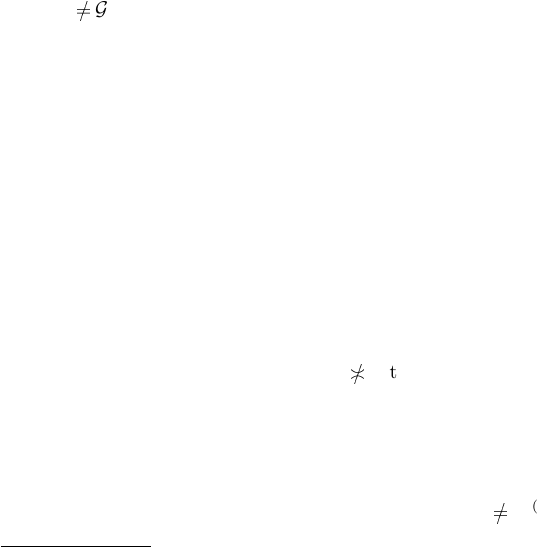Cauchy Filters on R eloi ds
by Victor Porton
Email: porton@narod.ru
Web: http://www.mathematics21.org
March 7, 2014
Abstract
In this article I consider low lters on reloids, generalizing Cauchy lters on uniform spaces.
Using low lters, I dene Cauchy-complete reloids, generalizing complete uniform spaces.
1 Preface
This is a preliminary partial draft.
To understand this article you need ﬁrst look into my book .
As my book is yet in preprint stage and I may change it, I probably will integrate the content
http://math.stackexchange.com/questions/401989/what-are-interesting-properties-of- totally-
bounded-uniform-spaces
http://ncatlab.org/nlab/show/proximity+space#uniform_spaces for a proof sketch that prox-
imities correspond to totally bounded uniformi ties.
2 Low ﬁlters space
Deﬁnition 1. A lower set
1
of proper ﬁlters on U (a set) is a set C of proper ﬁlters on U, such
that if 0
G F and F C then G C . [TODO: Probably should include the improper ﬁlter.]
Deﬁnition 2. I call low ﬁlters space a set together with a lower set of proper ﬁlters on this set.
Deﬁnition 3. PR(U; C ) = C ; Ob(U ; C ) = U .
2
Deﬁnition 4. Introduce an order on low ﬁlters spaces: (U; C ) (U ; D ) C D .
3 Cauchy spaces
Deﬁnition 5. A Cauchy space on a set X is a low ﬁlters space (U; C ) (element of C are called
Cauchy ﬁlters) such that:
1. x U :
X
{x} C ;
2. If F, G are Cauchy ﬁlters and F
G then F G is a Cauchy ﬁlter.
Deﬁnition 6. A completely Cauchy space on a set X is a low lters space (U; C ) (element of C
are called Cauchy lters) such that:
1. x X:
X
{x} C ;
2. If S is a nonempty set of Cauchy ﬁlters and
d
S
0
F(X)
then
F
S is a Cauchy ﬁlter.
1. Remember that our orders on lters is the reverse to set theoretic inclusion. It could be called an upper set
in other sources.
2. PR is from English word prole.
1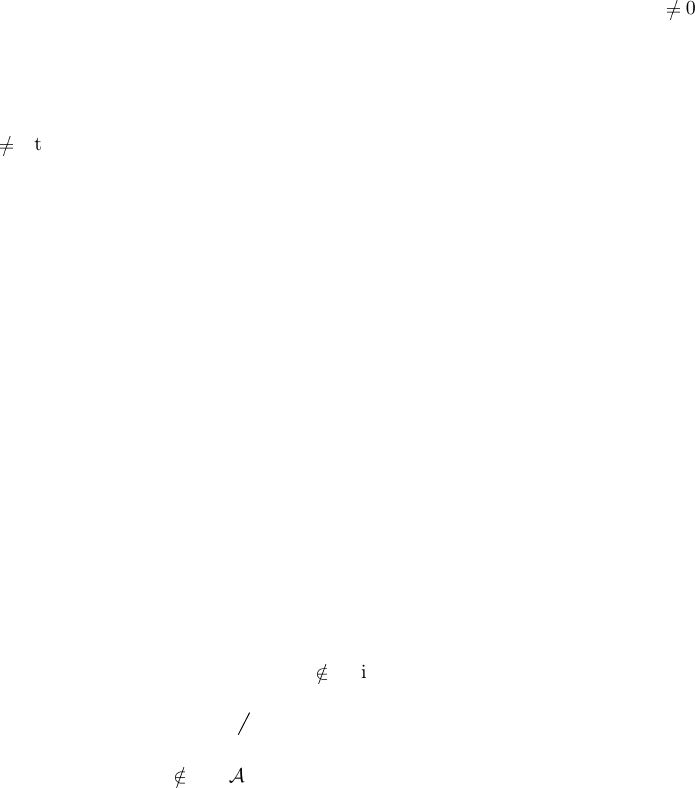Obvi ous 7. Every completely Cauchy space is a Cauchy space.
Proposition 8.
F
{X C | X F }
S =
F
S for nonempty S P {X C | X F }, provided that F
is a xed Cauchy ﬁlter on a completely Cauchy space.
Proof. F is proper. So for every nonempty S P {X C | X F } we have
d
S F
0
F(X)
.
Thus
F
S is a Cauchy ﬁlter and so
F
S {X C | X F }.
Proposition 9. If F is a ﬁxed Cauchy ﬁlte r on a completely Cauchy space, then the poset
{X C | X F } (wit h th e induced order) is a complete lattice.
Proof. If S
then
F
{X C | X F }
S =
F
S. If S = then
F
{X C | X F }
S = F.
Corollary 10. If F is a ﬁxed Cauchy ﬁlter on a completely Cauchy space, then the poset {X
C | X F } (with the induced order) has a maximum.
4 Relationships with s ymmetri c reloids
Deﬁnition 11. Denote (RLD)
Low
(U ; C ) =
F
{X ×
RLD
X | X C }.
Deﬁnition 12. (Low)ν (low ﬁlters for reloid ν) is a l ow ﬁlters space on U such that
PR (Low)ν = {X F
U
\ {0
F
} | X ×
RLD
X ν }.
Theorem 13. If (U ; C ) is a low ﬁlters space, then (U ; C ) = (Low)(RLD)
Low
(U ; C ).
Proof. If X C then X ×
RLD
X (RLD)
Low
(U; C ) a nd thus X PR (Low)(RLD)
Low
(U; C ). Thus
(U ; C ) (Low)(RLD)
Low
(U; C ).
Let’s prove (U; C ) (Low)(RLD)
Low
(U; C ).
Let A PR (Low)(RLD)
Low
(U ; C ). We need to prove A C .
Really A ×
RLD
A (RLD)
Low
(U; C ). It is enough to prove that ∃X C : A X .
Suppose X C : A X .
For every X C obtain X
X
X such that X
X
A (if forall X X we have X
X
A, then X A
what is contrary to our supposition).
It is now enough to prove A ×
RLD
A
F
{↑
U
X
X
×
RLD
U
X
X
| X C }.
Really,
F
{↑
U
X
X
×
RLD
U
X
X
| X C } =
RLD(U ;U)
S
{X
X
× X
X
| X C }. So our claim takes
the form
S
{X
X
× X
X
| X C }
GR(A ×
RLD
A) that is A A:
S
{X
X
× X
X
| X C } + A × A
what is true be cause X
X
+ A for ever y A A.
Remark 14. The last theorem does not hold with X ×
FCD
RLD
X (take C =
{{x} | x U } for an inﬁnite set U as a counter-example).
Remark 15. Not every symmetric reloid is in the form (RLD)
Low
(U; C ) for some Cauchy space
(U ; C ). The same Cauchy space can be induced by diﬀerent uniform spaces. Se e http://math.stack-
exchange.com/questions/702182/diﬀerent-uniform-spaces-having-the-same-set-of-cauchy-ﬁlters
[TODO: Is composition of two images of low ﬁlter spaces also a low ﬁlters space?]
5 More on Cauchy ﬁlt ers
Obvi ous 16. Low ﬁlter on an endoreloid ν is a ﬁlter F such that
U GR f A F: A × A U .
Remark 17. The above formula is the standard deﬁnition of Cauchy ﬁlters on uniform spaces.
2 Section 5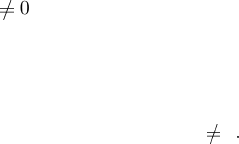Proposition 18. If ν ν ν
1
then every neighborhood ﬁlter is a Cauchy ﬁlter, that it
ν h(FCD)ν i
{x} ×
RLD
h(FCD)νi
{x}
for every point x.
Proof. h(FCD)ν i
{x} ×
RLD
h(FCD)νi
{x} = h(FCD)ν i↑
Ob ν
{x} ×
RLD
h(FCD)νi↑
Ob ν
{x} = ν
(
Ob ν
{x} ×
RLD
Ob ν
{x}) ν
1
= ν
RLD(Ob ν;Ob ν)
{(x; x)}
ν
1
ν id
RLD(Ob ν;Ob ν)
ν
1
=
ν ν
1
ν.
Proposition 19. If a ﬁlte r converges to a point, it is a low ﬁlter, provided that every neighborhood
ﬁlter is a low ﬁlter.
Proof. Let F h(FCD)ν i
{x}. T hen F ×
RLD
F h(FCD)ν i
{x} ×
RLD
h(FCD)νi
{x} ν.
Corollary 20. If a ﬁlter converges to a po int, it is a low lter, provided that ν ν ν
1
.
6 Maximal Cauchy ﬁlters
Lemma 21. Let S be a set of sets with
d
h↑
F
iS
0
F
(in other words, S has ﬁnite intersection
property). Let T = {X × X | X S }. Then
[
T
[
T =
[
S ×
[
S.
Proof. Let x
S
S. Then x X for some X S. h
S
T i{x} X
T
S
. Thus
h
S
T
S
T i{x} = h
S
T ih
S
T i{x} h↑
FCD
S
T i
d
h↑
F
iS
F
{h↑
FCD
(X × X)i
d
h↑
F
iS | X
S } =
F
{↑
F
X | X S } =
F
h↑
F
iS that is h
S
T
S
T i{x}
S
S.
Corollary 22. Let S be a set of ﬁlters (on some ﬁxed set) with nonempty meet. Let
T = {X ×
RLD
X | X S }
Then
G
T
G
T =
G
S ×
RLD
G
S.
Proof.
F
T
F
T =
d
{↑
F
(X X) | X
F
T }.
If X
F
T then X =
S
QT
(P
Q
× P
Q
) where P
Q
Q. Therefore by the lemma we have
[
{P
Q
× P
Q
| Q T }
[
{P
Q
× P
Q
| Q T } =
[
QT
P
Q
×
[
QT
P
Q
.
Thus X X =
S
QT
P
Q
×
S
QT
P
Q
.
Consequently
F
T
F
T =
d
F
S
QT
P
Q
×
S
QT
P
Q
| X
F
T
F
S ×
RLD
F
S.
F
T
F
T
F
S ×
RLD
F
S is obvious.
Deﬁnition 23. I call an endorelo id ν symmetrically tra nsitive iﬀ for every symmetric endofuncoid
f FCD(Ob ν; Ob ν) we have f ν f f ν.
Obvi ous 24. It is symmetrically transitive if at le ast one of the following holds:
1. ν ν ν;
2. ν ν
1
ν;
3. ν
1
ν ν.
4. ν
1
ν
1
ν.
Corollary 25. Every uniform space is symmetrically transitive.
Maximal Cauchy filters 3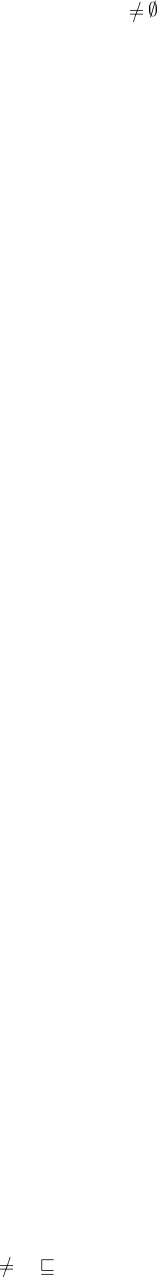Proposition 26. (Low)ν is a completely Ca uchy space for every symmetrically transitive
endoreloid ν.
Proof. Suppose S P {X F \ {0
F
} | X ×
RLD
X ν } and S
.
F
{X ×
RLD
X | X S} ν;
F
{X ×
RLD
X | X S}
F
{X ×
RLD
X | X S} ν;
F
S ×
RLD
F
S ν (taken into account that S has nonempty meet). Thus
F
S is Cauchy.
Proposition 27. The neighbourhood ﬁlter h(FCD)ν i
{x} of a point x Ob ν is a maximal Cauchy
ﬁlter, if it is a Cauchy ﬁlter and ν is a reﬂexive reloid.
[TODO: Does it holds for all low ﬁlters?]
Proof. Let N = h(FCD)ν i
{x}. Let C N be a Cauchy ﬁlter. We need to s how N C .
Since C is Cauchy ﬁlter, C ×
RLD
C ν. Since C N we have C is a neighborhood of x and thus
Ob ν
{x} C (reﬂexivity of ν). Thus
Ob ν
{x}×
RLD
C C ×
RLD
C and hence
Ob ν
{x} ×
RLD
C ν;
C im(ν |
Ob ν
{x}
) = h(FCD)ν i
{x} = N .
7 Cauchy continuous functions
Deﬁnition 2 8. A function f : U V is Cauchy continuous from a low ﬁlters space (U; C ) to a
low ﬁlters space (V ; D) when ∀X C : h↑
FCD
f iX D .
Proposition 29. Let f is a pr incipal reloid. Then f C((RLD)
Low
C ; (R LD )
Low
D) iﬀ f is Cauchy
continuous.
f (RLD)
Low
C f
1
(RLD)
Low
D
G
{f (X ×
RLD
X ) f
1
| X C } (RLD)
Low
D
G
{h↑
FCD
f iX ×
RLD
h↑
FCD
f iX | X C } (RLD)
Low
D
∀X C : h↑
FCD
f iX ×
RLD
h↑
FCD
f iX (RLD)
Low
D
∀X C : h
FCD
f iX D .
Thus we have expres sed Cauchy properties through the algebra of reloids.
8 Cauchy-complete relo ids
Deﬁnition 30. An endoreloid ν is Ca uchy-complete iﬀ every low ﬁlter for this reloid converges to
a point.
Remark 31. In my book  comple te reloid means something diﬀerent. I will always prepend the
word “Cauchy” to the word “complete” whe n meaning is by the last deﬁnition.
https://en.wikipedia.org/wiki/Complete_uniform_space#Completeness
9 Totally bounded
http://ncatlab.org/nlab/show/Cauchy+space
Deﬁnition 32. Cauchy space is called totally bounded when every proper ﬁlter contains a Cauchy
ﬁlter.
Obvi ous 33. A re loid ν is to tally bounded iﬀ
X P Ob ν ∃X F
Ob ν
: (0
X
Ob ν
X X ×
RLD
X ν).
4 Section 9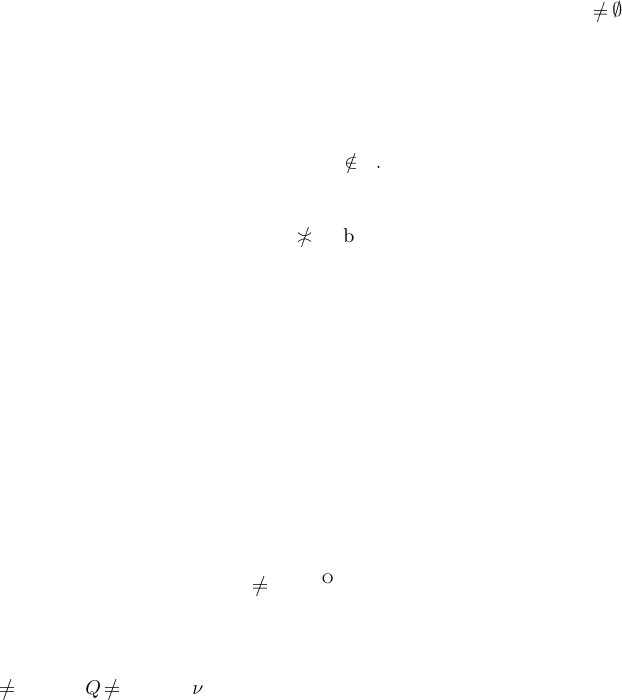Theorem 34. A symmetric transitive reloid is totally bounded iﬀ its Cauchy space is totally
bounded.
Proof.
. Let F be a proper ﬁlter on Ob ν and let a atoms F. It’s enough to prove that a is Cauchy.
Let D GR ν. Let also E GR ν is symmetric and E E D. There existsa ﬁnite subset
F Ob ν such that hE iF = Ob ν. Then obvio usly exists x F such that a
Ob ν
hE i{x},
but hE i{x} × hE i{x} = E
1
({x} × {x}) E D, thus a ×
RLD
a
RLD(Ob ν;Ob ν)
D.
Because D was taken arbit rary, we have a ×
RLD
a ν that is a is Cauchy.
. Suppose that Cauchy space associated with a reloid ν is totally bounded but the reloid
ν isn’t totally bounded. So the re exists a D GR ν such that (Ob ν) \ hDiF
for every
ﬁnite set F .
Consider the ﬁlter base
S = {(Ob ν) \ hD iF | F P Ob ν , F is ﬁnite}
and the ﬁlter F =
d
h↑
Ob ν
iS generated by this base. The ﬁlter F is proper be cause
intersection P Q S for every P , Q S and
S. Thus there exists a Cauchy (for our
Cauchy space) ﬁlter X F that is X ×
RLD
X ν.
Thus there exists M X such that M × M D . Let F be a ﬁnite subset of Ob ν.
Then (Ob ν) \ hDiF F X . Thus M
(Ob ν) \ hDiF and so there exists a point
x M ((Ob ν) \ hDiF ).
hM × M i{p} hDi{x} for every p M; thus M hDi{x}.
So M hD i(F {x}). But this means that M X does not intersect (Ob ν) \
hDi(F {x}) F X , what is a contradiction (taken into account that X is proper).
http://math.stackexchange.com/questions/104696/pre-compactness-total-boundedness-and-
cauchy-sequential-compactness
10 Totally bounded funcoids
Deﬁnition 35. A funcoid ν is totally bounde d iﬀ
X Ob ν X F
Ob ν
: (0
X
Ob ν
X X ×
FCD
X ν).
This can be rewritten in elementary terms (without using fu nc oidal product:
X ×
FCD
X ν P X : X hν iP P X , Q X : P [ν]
Q P , Q Ob ν:
(E X : (E P
E Q
) P [ν]
Q).
Note that probably I am the ﬁrst p e rson which has written the above formula (for p roximity
spaces for instance) explicitly.
11 On principal low lter spaces
Deﬁnition 36. A low ﬁlter sp ace (U ; C ) is principal when all ﬁlters in C are principal.
Deﬁnition 37. A low ﬁlter sp ace (U ; C ) is reﬂexive when x U :
U
{x} C .
Proposition 38. Having ﬁxed a set U , principal reﬂexive low ﬁlter spaces on U bijectively cor-
respond to principal reﬂexive symmertic endoreloids on U.
Proof. ??
http://math.stackexchange.com/questions/701684/union-of-cartesian-squares
On principal low filter spaces 5
12 Rest
https://en.wikipedia.org/wiki/Cauchy_ﬁlter#Cauchy_ ﬁlters
https://en.wikipedia.org/wiki/Uniform_space “Hausdorﬀ completion of a uniform space” here)
http://at.yorku.ca/z/a/a/b/13.htm : the category Prox of proximity spaces and proximally
continuous maps (i.e. maps preserving nearness between two sets) is isomorphic to the category
of totally bounded uniform spaces (and uniformly continuous maps).
https://en.wikipedia.org/wiki/Cauchy_space http://ncatlab.org/nlab/show/Cauchy+space
http://arxiv.org/abs/1309.1748